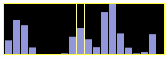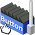## 28 March 2009

### Chart in Javascript

There aren't enough bar-chart-drawing implementations out there yet, so this article will present the best one yet. It looks like this:Or several little charts in a row, like this:Features:

• Really easy to use
• mouse move highlights bar under mouse
• pops up tooltip with information for the bar under the mouse
• All colours are configurable
• Scales data automatically to height of canvas
• Calculates thickness of bars automatically so all fit in the width of the canvas
• Redraw data as often as you want - for example with data from an ajax call

The tooltip is missing from this screenshot. My mac hides the tooltip before it takes the screenshot unfortunately.

Anyway, I'd like to convince you that it's really, really easy to use. Supply your data in JSON format like this:

```var data = [
[ "week 1", "1000" ],
[ "week 2", "2000" ],
[ "week 3", "3000" ]
];
```

Declare a `canvas` element:

```<canvas id="weekly_chart" width="200" height="80"></canvas>
```

Then just call the `Chart(canvas_element, bar_colour, bg_colour, hilite_colour)` constructor:

```var chart = new Chart(\$('weekly_chart'), "rgb(128,128,255)", "rgb(0,0,0)", "rgb(255,255,128)");
```

```chart.draw(data);
```

It couldn't be simpler!

```(function() {
function max(data) {
var max = 0;
for (var i = 0; i < data.length; i++) {
if (data[i] > max) {
max = data[i];
}
}
return max;
}

function coords(event, element, f) {
var offset = \$(element).cumulativeOffset();   // \$ from prototype
var p = Event.pointer(event || window.event); // Event.pointer from prototype
var y = p.y - offset.top;
var x = p.x - offset.left;
f(y, x);
}

window.Chart = function(canvas, fg, bg, hilite) {
if (!canvas || !canvas.getContext) {
return;
}

var cx = canvas.getContext('2d');

this.draw = function(data) {
cx.clearRect(0, 0, canvas.width, canvas.height);
var thick = canvas.width / data.length;
var scale = canvas.height / max(data);

function highlightBars(index) {
cx.lineWidth = 1;

for (var i = 0; i < data.length; i++) {
if (i == index || i == (index + 1)) {
cx.strokeStyle = hilite;
} else {
cx.strokeStyle = bg;
}
cx.beginPath();
cx.moveTo((i * thick) + 0.5, 0);
cx.lineTo((i * thick) + 0.5, canvas.height);
cx.stroke();
}
}

cx.fillStyle = bg;
cx.fillRect(0, 0, canvas.width, canvas.height);

cx.fillStyle = fg;

for (var i = 0; i < data.length; i++) {
var h = data[i] * scale;
if (!isNaN(h)) {
cx.fillRect(i * thick, canvas.height - h, thick, h);
}
}

highlightBars(-2);

canvas.onmouseout = function(event) {
highlightBars(-2);
};

canvas.onmousemove = function(event) {
coords(event, canvas, function(y, x) {
var index = ((x-1) / thick).floor();
if (index < 0) {
index = 0;
}
highlightBars(index);

var bar = data[index];
if (bar) {
canvas.title = bar + " : " + bar;
}
});
};
};
};
})();
```

This is the simple version. Feel free to comment with improvements, I'll keep the code up to date with my favourite suggestions.

1.Internet Exploder ;-)

2.Conan,

Do you know about Google Chart? It's a web service for generating charts (in png format).

You can do a lot of complicated things with it, but in the same time the API is quite simple.